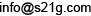# API

## To get a mathematical expression into an image

The formula service provides an API to get mathematical expressions into images in the simple manner below.

### SYNOPSIS

 http://formula.s21g.com/?{expr}.png 

Where expr is a mathematical expression in LaTeX format. For example,

 http://formula.s21g.com/f(x)=\int_0^{x}g(t)\,dt.png 

You can get the image below if you request the URI above from a HTML document.Typically the request is caused from IMG tag, like this

<img src=http://formula.s21g.com/?f(x)=\int_0^{x}g(t)\,dt.png" />
<img src=http://formula.s21g.com/?f(x)=\int_0%5E%7Bx%7Dg(t)\,dt.png" />

The expr can be escaped as an URI parameter.

### Requirements

This API has several requirements to use.

• You need to set a correct HTTP_REFERER to each request. The referer should be an URI of an existing HTML document. The document should be accessible from the servers of this service.
• You need to enclose the IMG tag which requests a mathematical expression to this service with the A tag which links to the page of the expression, like this.

<a href="http://formula.s21g.com/?f(x)=\int_0^{x}g(t)\,dt">
<img src="http://formula.s21g.com/?f(x)=\int_0^{x}g(t)\,dt.png" />
</a>

The URI of the page is partially same to the request's URI except not including .png at the end.

You can have other attributes in the tags as long as the attributes required are provided.

• We can't promise the service is always working well because of being provided as free. If you need more stability, please contact us by email toor twitter to @takiuchi.

And we appreciate any kind of feedbacks. Feel free to contact us.